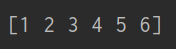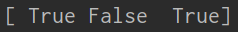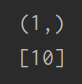# Attributeerror: numpy.ndarray object has no attribute values

If you have been working with NumPy, you may have come across the AttributeError: numpy.ndarray object has no attribute values error message.

This error occurs when you try to access the “values” attribute of a NumPy ndarray object, but it does not exist.

In this article, we will discuss what is this error and how to fix it.

We will also provide some example codes for avoiding similar errors in the future.

Before we dive into the error, it’s important to understand what a NumPy ndarray is…

## What is Numpy nd.array?

A ndarray is a multidimensional array of elements of the same type.

It is the core data structure used in NumPy.

You can create a NumPy ndarray using the numpy.array() function.

For example:

```import numpy as np

arr = np.array([1, 2, 3])```

This creates a one-dimensional ndarray with the values:

[1, 2, 3]

You can also create multi-dimensional ndarrays using nested lists.

For example:

arr = np.array([[1, 2, 3], [4, 5, 6]])

This creates a two-dimensional ndarray with the values:

[[1, 2, 3], [4, 5, 6]]

Now that we understand what a NumPy ndarray is, let’s look at the “AttributeError: numpy.ndarray object has no attribute values” error.

## What is attributeerror: numpy.ndarray object has no attribute values?

This attributeerror: numpy.ndarray object has no attribute values error occurs when you try to access the “values” attribute of a NumPy ndarray object, but it does not exist.

The “values” attribute is not a built-in attribute of ndarrays, so it is not available to be accessed directly.

Here is an example:

```import numpy as np

arr = np.array([1, 2, 3])
arr.values```

This code will result in the following error message:

AttributeError: ‘numpy.ndarray’ object has no attribute ‘values’

## How to fix attributeerror: numpy.ndarray object has no attribute values

Here are some solutions to fix the numpy.ndarray object has no attribute values error:

1. Solution 1: Use numpy.array.tolist()

One way to fix this error is to convert the NumPy array to a list using the numpy.array.tolist() method.

This method will convert the NumPy array to a list, which has a values attribute that can be accessed.

import numpy as np

#Create a NumPy array
arr = np.array([1, 2, 3, 4, 5])

#Convert the NumPy array to a list
lst = arr.tolist()

#Access the values attribute of the list
print(lst)

Output:2. Solution 2: Use numpy.ndarray.flatten()

Another way to fix this error is to use the numpy.ndarray.flatten() method, which returns a flattened version of the NumPy array.

The flattened version is a 1D array that has a values attribute that can be accessed.

import numpy as np

#Create a NumPy array
arr = np.array([[1, 2], [3, 4], [5, 6]])

#Flatten the NumPy array
flat_arr = arr.flatten()

#Access the values attribute of the flattened array
print(flat_arr`)`

Output:3. Solution 3: Check the data type of the NumPy array

If the above solutions do not work, you should check the data type of the NumPy array.

The values attribute may not be available for some data types, such as bool or int.

import numpy as np

#Create a NumPy array with the data type 'bool'
arr = np.array([True, False, True])

#Try to access the values attribute
print(arr)

Output:4. Solution 4: Check the shape of the NumPy array

The shape of a NumPy array specifies the number of elements along each dimension.

If the NumPy array has a single element, it may not have the values attribute.

In this case, you can convert the NumPy array to a list and then access the values attribute of the list.

import numpy as np

#Create a NumPy array with a single element
arr = np.array()

#Check the shape of the NumPy array
print(arr.shape) # Output: (1,)

#Convert the NumPy array to a list and access the values attribute
lst = arr.tolist()
print(lst)

Output:## Conclusion

The numpy.ndarray object has no attribute values error can be frustrating, but it can be fixed using the solutions discussed in this article.

You can convert the NumPy array to a list, flatten it, check its data type, or check its shape to access the values attribute.

Remember to use the appropriate solution depending on the cause of the error.

We hope that this article has provided you with the information you need to fix this error and continue working with Python.

If you are finding solutions to some errors you’re encountering we also have Modulenotfounderror: no module named ‘ipython’.